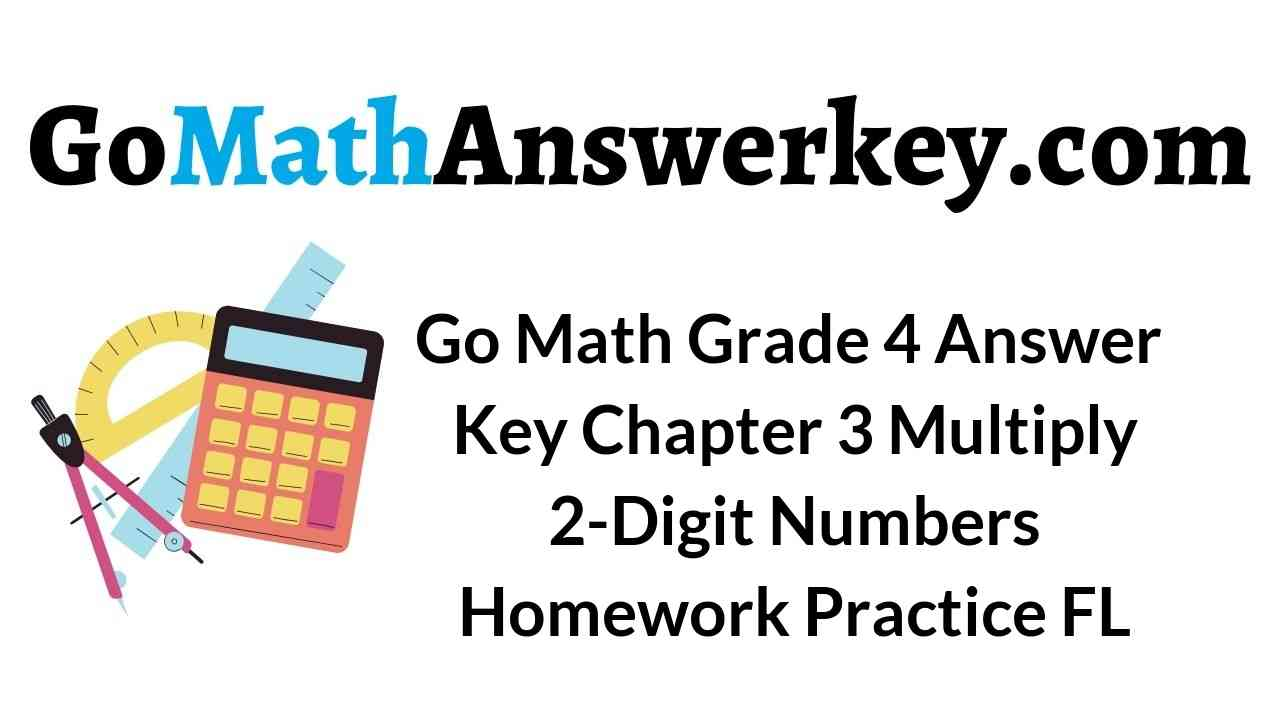# Go Math Grade 4 Answer Key Homework Practice FL Chapter 3 Multiply 2-Digit Numbers

Go Math Grade 4 Answer Key Homework Practice FL Chapter 3 Multiply 2-Digit Numbers helps the parents to make their child understand the concepts of multiplication. It is very important for students to learn the fundamentals in primary schools. Or else you will face many issues in the secondary standards. We help the students to overcome the difficulties of basics of maths by providing the Answer Key for Go Math Grade 4 Homework Practice FL Chapter 3 Multiply 2-Digit Numbers.

## Go Math Grade 4 Answer Key Homework Practice FL Chapter 3 Multiply 2-Digit Numbers

Refer Go Math Grade 4 Answer Key Homework Practice FL Chapter 3 Multiply 2-Digit Numbers by downloading the pdf for free. We have presented the step by steps explanation for all the questions here. All you have to do is to click on the below links and get the simple techniques to solve the solutions.

Lesson: 1 – Multiply by Tens

Lesson: 2 – Estimate Products

Lesson: 3 – Investigate • Area Models and Partial Products

Lesson: 4 – Multiply Using Partial Products

Lesson: 5 – Multiply with Regrouping

Lesson: 6 – Choose a Multiplication Method

Lesson: 7 – Problem Solving • Multiply 2-Digit Numbers

Lesson: 8

### Common Core – Multiply 2-Digit Numbers – Page No. 51

Multiply by Tens

Choose a method. Then find the product.

Question 1.
16 × 60 = 960
Use the halving-and-doubling strategy.
Find half of 16: 16 ÷ 2 = 8.
Multiply this number by 60: 8 × 60 = 480
Double this result: 2 × 480 = 960

Question 2.
80 × 22 = _____

Explanation:
By using the place value method, Multiply 80 × 22
You can think of 80 as 8 tens
80 × 22 = (22 × 8) tens
= 176 tens
= 176 × 10 = 1760
80 × 22 = 1760

Question 3.
30 × 52 = _____

Explanation:
Use the Associative Property
You can think of 30 as 3 × 10
30 × 52 = (3 × 10) × 52
= 3 × (10 × 52)
= 3 × 520
= 1560
30 × 52 = 1560

Question 4.
60 × 20 = _____

Explanation:
60 × 20
Use the halving and doubling strategy
half of the 60 to make the problem simpler
60/ 2 = 30
Multiply 30 with 20
30 × 20 = 600
Double the 600
2 × 600= 1200
60 × 20 = 1200

Question 5.
40 × 35 = _____

Explanation:
By using the Associative Property 40 × 35
You can think of 40 as 4 × 10
40 × 35 = (4 × 10) × 35
= 4 × (10 × 35)
= 4 × 350
= 1400
40 × 35 = 1400

Question 6.
10 × 90 = _____

Explanation:
By using the place value method, Multiply 10 x 90
You can think of 90 as 9 tens
10 × 90 = (10 × 9) tens
= 90 tens
= 10 × 90 = 900

Question 7.
31 × 50 = _____

Explanation:
Use the place value method to multiply 31 × 50
You can think of 50 as 5 tens
31 × 50 = 31 × 5 tens
= 155 tens
= 1,550
31 × 50 = 1,550

Problem Solving

Question 8.
Kenny bought 20 packs of baseball cards. There are 12 cards in each pack. How many cards did Kenny buy?
_____ cards

Explanation:
From the given data,
Kenny bought 20 packs of basketball cards
There are 12 cards in each pack = 12 × 20 cards
Use the associative property
You can write 20 as 2 × 10
12 × 20 = 12 × (2 × 10)
= (12 × 2) × 10
= (24) × 10
= 240 cards
Kenny bought 240 cards

Question 9.
The Hart family drove 10 hours to their vacation spot. They drove an average of 48 miles each hour. How many miles did they drive in all?
_____ miles

Explanation:
As per the given data,
Hart family drove 10 hours to their vacation spot
The average speed per each hour is = 48 miles
Total miles = 48 × 10
Use the halving and doubling strategy
Half of the 48 to make the problem simpler
48/ 2 = 24
Multiply 24 with 10 = 24 × 10 = 240
Double the value = 2 × 240 = 480 miles
Total miles drove by hart family = 480 miles.

### Common Core – Multiply 2-Digit Numbers – Page No. 52

Lesson Check

Question 1.
For the school play, 40 rows of chairs are set up. There are 22 chairs in each row. How many chairs are there in all?
Options:
a. 800
b. 840
c. 880
d. 8,800

Explanation:
As per the given data
For the school play, 40 rows of chairs are available. 22 chairs are available in each row.
Then total chairs in school play are = 22 × 40
By using the place value method
You can think of 40 as 4 tens
22 × 40 = 22 × 4 tens
= 88 tens
= 880
Total chairs in school are = 880
Thus the answer is option c.

Question 2.
At West School, there are 20 classrooms. Each classroom has 20 students. How many students are at West School?
Options:
a. 40
b. 400
c. 440
d. 4,000

Explanation:
From the given data,
Total classrooms in west school = 20
Number of students per each classroom = 20
Then, total students at West School = 20 × 20
By using the associative property
You can think of 20 as 2 × 10
20 × 20 = 20 × (2 × 10)
= (20 × 2) × 10
=(40) × 10
=400
Total number of students at West School = 400
Thus the answer is option b.

Spiral Review

Question 3.
Alex has 48 stickers. This is 6 times the number of stickers Max has. How many stickers does Max have?
Options:
a. 6
b. 7
c. 8
d. 9

Explanation:
As per the give data,
Alex has 48 stickers
That means, X= 48
This is 6 times the number of stickers max has = Y = 6X = 48
Then, number of stickers with Max = Y = X = 48/6 = 8
Number of stickers with Max = Y = 8 Stickers.
Thus the answer is option c.

Question 4.
Ali’s dog weighs 8 times as much as her cat. Together, the two pets weigh 54 pounds. How much does Ali’s dog weigh?
Options:
a. 6 pounds
b. 42 pounds
c. 46 pounds
d. 48 pounds

Explanation:
From the given data,
Ali’s cat weight = X
Ali’s dog weight = 8 times as much as Ali’s cat = 8X
Together, the two pets weight = (X + 8X) = 54 pounds
9X = 54 pounds
X = 54/9 pounds = 6 pounds
Then, Ali’s dog weight = 8X =8 x 6 = 48 pounds.
Thus the answer is option d.

Question 5.
Allison has 3 containers with 25 crayons in each. She also has 4 boxes of markers with 12 markers in each box. She gives 10 crayons to a friend. How many crayons and markers does Allison have now?
Options:
a. 34
b. 113
c. 123
d. 133

Explanation:
As per the given data,
Allison has 3 containers with 25 crayons in each = X = 3 × 25 = 75
Allison has 4 boxes of markers with 12 markers in each box = Y = 4 × 12 = 48
Allison gives 10 crayons to a friend = Z = 75 – 10 = 65
Now, total number of crayons and markers with Allison = Y + Z = 48 + 65 = 113
Thus the answer is option b.

Question 6.
The state of Utah covers 82,144 square miles. The state of Montana covers 145,552 square miles. What is the total area of the two states?
Options:
a. 63,408 square miles
b. 223,408 square miles
c. 227,696 square miles
d. 966,992 square miles

Explanation:
From the given data,
The state of Utah covers 82,144 square miles
The state of Montana covers 145,552 square miles
Then, Total area of the two states = 82,144 + 145,552
The total area of two states = 227,696 square miles.
Thus the answer is option c.

### Common Core – Multiply 2-Digit Numbers – Page No. 53

Estimate Products

Estimate the product. Choose a method.

Question 1.
38 × 21
38 × 21
↓        ↓
40 × 20
800

Question 2.
63 × 19
Estimate: _______

Explanation:
63     19
↓        ↓
60     20
Use mental math
60 × 20 = 1200

Question 3.
27 × $42 Estimate: _______ Answer: 1200 Explanation: 27 42 ↓ ↓ 30 40 Use mental math 30 ×$40 = $1200 Question 4. 73 × 67 Estimate: _______ Answer: 4900 Explanation: 73 6770 70 Use mental math 70 × 70 = 4900 Question 5. 37 ×$44
Estimate: _______

Explanation:
37     44
↓        ↓
40     40
Use mental math
40 × $40 =$1600

Question 6.
85 × 71
Estimate: _______

Explanation:
85     71
↓        ↓
90     70
Use mental math
90 × 70 = 6300

Question 7.
88 × 56
Estimate: _______

Explanation:
88     56
↓        ↓
90      60
Use mental math
90 × 60 = 5400

Question 8.
97 × 13
Estimate: _______

Explanation:
97     13
↓        ↓
100    10
Use mental math
100 × 10 = 1000

Question 9.
92 × 64
Estimate: _______

Explanation:
92     64
↓        ↓
90      60
Use mental math
90 × 60 = 5400

Problem Solving

Question 10.
A dime has a diameter of about 18 millimeters. About how many millimeters long would a row of 34 dimes be?

Explanation:
From the given data,
A dime has a diameter of about 18 millimeters
Then, 34 dimes diameter = 18 × 34
18 x 34
↓        ↓
20 × 30 = 600
So, 34 dimes have a diameter of about 600 millimeters long

Question 11.
A half-dollar has a diameter of about 31 millimeters. About how many millimeters long would a row of 56 half-dollars be?

Explanation:
As per the given data,
A half – dollar has a diameter of about 31 millimeters
Then, 56 half-dollars diameter = 31 × 56
31     56
↓        ↓
30     60
So, 56 half-dollars have a diameter of about 1800 millimeters long.

### Common Core – Multiply 2-Digit Numbers – Page No. 54

Lesson Check

Question 1.
Which is the best estimate for the product
43 × 68?
Options:
a. 3,500
b. 2,800
c. 2,400
d. 280

Explanation:
Round to the nearest tens
43 × 68
↓        ↓
40 × 70
Use mental math
4 × 7 = 28
40 × 70 = 2800
Estimated product of 43 × 68 = 2800
The correct answer is option b.

Question 2.
Marissa burns 93 calories each time she plays fetch with her dog. She plays fetch with her dog once a day. About how many calories will Marissa burn playing fetch with her dog in 28 days?
Options:
a. 4,000
b. 2,700
c. 2,000
d. 270

Explanation:
From the given data,
Marissa burned calories each time when she plays fetch with her dog= 93 calories
Then, Marissa burned calories in 28 days while playing fetch with her dog = 28 × 93
Round to the nearest tens
28 × 93
↓        ↓
30 × 90
Then, estimated burned calories in 28 days by Marissa = 2700 calories
The correct answer is option b.

Spiral Review

Question 3.
Use the model to find 3 × 126.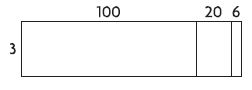Options:
a. 368
b. 378
c. 468
d. 478

Explanation:
From the above Figure,
3 × 126 = 3 × 100 + 3 × 20 + 3 × 6
= 300 + 60 + 18
= 378
3 × 126 = 378
The correct answer is option b.

Question 4.
A store sells a certain brand of jeans for $38. One day, the store sold 6 pairs of jeans of that brand. How much money did the store make from selling the 6 pairs of jeans? Options: a.$188
b. $228 c.$248
d. $288 Answer:$228

Explanation:
As per the given data,
A store sells a certain brand of jeans for rupees = $38 One day, the store sold 6 pairs of jeans of that brand = 6 ×$38
6 × $38 =$228
The total amount of 6 pairs of jeans = $228 The correct answer is option b. Question 5. The Gateway Arch in St. Louis, Missouri, weighs about 20,000 tons. Which amount could be the exact number of tons the Arch weighs? Options: a. 31,093 tons b. 25,812 tons c. 17,246 tons d. 14,096 tons Answer: 17,246 tons Explanation: From the given data, The Gateway Arch in St.Louis, Missouri weight = about 20,000 tons From the available options, 17,246 tons is closer to 20,000 tons Then, the exact number of tons the Arch weighs = 17,246 tons The correct answer is option c. Question 6. Which is another name for 23 ten thousands? Options: a. 23,000,000 b. 2,300,000 c. 230,000 d. 23,000 Answer: 230,000 Explanation: As per the data, Another name for 23 ten thousands = 23 × 10,000 = 230,000 Another name for 23 ten thousand = 230,000 The correct answer is option c. ### Common Core – Multiply 2-Digit Numbers – Page No. 55 Area Models and Partial Products Draw a model to represent the product. Then record the product. Question 1. 13 × 42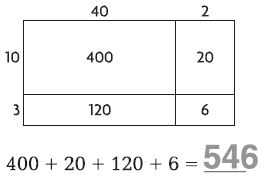Question 2. 18 × 34 = _____ Answer:300 + 40 + 240 + 32 = 612 Question 3. 22 × 26 = _____ Answer:400 + 120 + 40 + 12 = 572 Question 4. 15 × 33 = _____ Answer:300 + 30 + 150 + 15 = 495 Question 5. 23 × 29 = _____ Answer:400 + 180 + 60 + 27 = 667 Question 6. 19 × 36 = _____ Answer: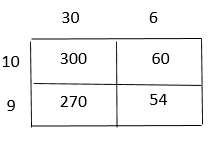300 + 60 + 270 + 54 = 684 Problem Solving Question 7. Sebastian made the following model to find the product 17 × 24.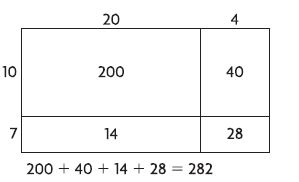Is his model correct? Explain. i. yes ii. no Answer: no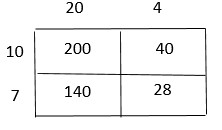200 + 40 + 140 + 28 = 408 Question 8. Each student in Ms. Sike’s kindergarten class has a box of crayons. Each box has 36 crayons. If there are 18 students in Ms. Sike’s class, how many crayons are there in all? _____ crayons Answer: 648 crayons Explanation: From the given information, Each student in Ms.Sike’s kindergarten class has a box of crayons Crayons in each box = 36 Crayons Number of students in Mr.Sike’s class = 18 students Total crayons = 18 × 36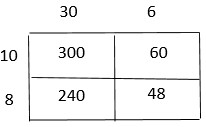300 + 60 + 240 + 48 = 648 ### Common Core – Multiply 2-Digit Numbers – Page No. 56 Lesson Check Question 1. Which product does the model below represent?Options: a. 161 b. 230 c. 340 d. 391 Answer: 391 Explanation: 200 + 30 + 140 + 21 = 391 17 × 23 = 391 The correct answer is option d. Question 2. Which product does the model below represent?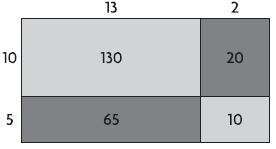Options: a. 219 b. 225 c. 244 d. 275 Answer: 225 Explanation: 130 + 20 + 65 + 10 = 225 15 × 15 = 225 The correct answer is option b. Spiral Review Question 3. Mariah builds a tabletop using square tiles. There are 12 rows of tiles and 30 tiles in each row. How many tiles in all does Mariah use? Options: a. 100 b. 180 c. 360 d. 420 Answer: 360 Explanation: From the given data, Mariah builds a tabletop using square tiles Square contains 12 rows of tiles and 30 tiles in each row = 12 × 30 12 × 30 = 360 tiles Total tiles used by Mariah = 360 tiles The correct answer is option c. Question 4. Trevor bakes 8 batches of biscuits, with 14 biscuits in each batch. He sets aside 4 biscuits from each batch for a bake sale and puts the rest in a jar. How many biscuits does Trevor put in the jar? Options: a. 112 b. 80 c. 50 d. 32 Answer: 80 Explanation: As per the given data, Number of biscuits baked by Trevor = 8 batches Number of biscuits in each batch = 14 biscuits So, total biscuits = 14 × 8 = 112 Trevor sets aside 4 biscuits from each batch for a bake = 8 × 4 = 32 biscuits are aside for a bake Trevor kept rest of biscuits in a jar = 112 – 32 = 80 So, 80 biscuits are put in the jar by Trevor. The correct answer is option b. Question 5. Li feeds her dog 3 cups of food each day. About how many cups of food does her dog eat in 28 days? Options: a. 60 cups b. 70 cups c. 80 cups d. 90 cups Answer: 80 cups Explanation: As per the given data, Li feeds her dog per day = 3 cups of food Then, Li feeds her dog for 28 days = 3 × 28 = 84 cups of food So, Li feeds her dog with 84 cups of food in 28 days. The correct answer is option c. Question 6. Which symbol makes the number sentence true? 4 ■ 0 = 0 Options: a. + b. – c. × d. ÷ Answer: × Explanation: 4 x 0 = 0 ### Common Core – Multiply 2-Digit Numbers – Page No. 57 Multiply Using Partial Products Record the product. Question 1. 2 3 × 7 9 1, 4 0 0 ——— 2 1 0 1 8 0 + 2 7 ——— 1, 8 17 Question 2. 5 6 × 3 2 ——— ________ Answer: 1,792 Explanation: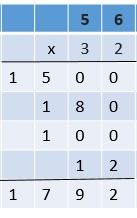Question 3. 8 7 × 6 4 ——— ________ Answer: 5,568 Explanation: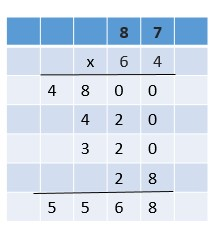Question 4. 3 3 × 2 5 ——— ________ Answer: 825 Explanation: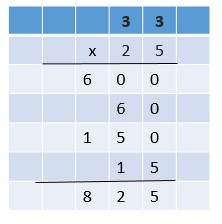Question 5. 9 4 × 1 2 ——— ________ Answer: 1,128 Explanation:Question 6. 5 1 × 7 7 ——— ________ Answer: 3,927 Explanation: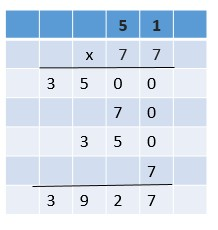Question 7. 6 9 × 4 9 ——— ________ Answer: 3,381 Explanation:Question 8. 8 6 × 8 4 ——— ________ Answer: 7,224 Explanation: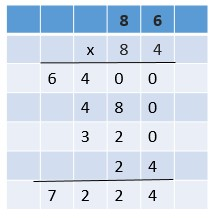Question 9. 9 8 × 4 2 ——— ________ Answer: 4,116 Explanation: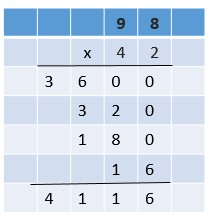Question 10. 7 3 × 3 7 ——— ________ Answer: 2,701 Explanation:Question 11. 8 5 × 5 1 ——— ________ Answer: 4,335 Explanation:Problem Solving Question 12. Evelyn drinks 8 glasses of water a day, which is 56 glasses of water a week. How many glasses of water does she drink in a year? (1 year = 52 weeks) ______ glasses Answer: 2,912 glasses Explanation: As per the given data, Evelyn drinks 8 glasses of water a day Evelyn drinks water per week = 56 glasses Then, the number of glasses per 52 weeks = 52 × 56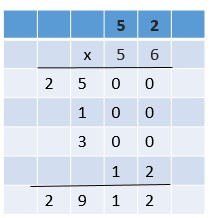Total number of glasses of water drink by Evelyn per year = 2912 glasses of water Question 13. Joe wants to use the Hiking Club’s funds to purchase new walking sticks for each of its 19 members. The sticks cost$26 each. The club has $480. Is this enough money to buy each member a new walking stick? If not, how much more money is needed? Is the money enough? ________ How much more is needed? ________ Answer: This amount is not enough to buy walking sticks Still,$14 amount is needed to buy walking sticks

Explanation:
From the given data,
Joe wants to use the Hiking club funds to purchase new walking sticks for each of its 19 members
Cost per each stick = $26 Total walking sticks cost per 19 members =$26 × 19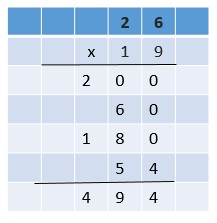Total cost for walking sticks for 19 members = $494 The club has =$480
This amount is not enough to buy walking sticks.
Still, $14 amount is needed to buy walking sticks. ### Common Core – Multiply 2-Digit Numbers – Page No. 58 Lesson Check Question 1. A carnival snack booth made$76 selling popcorn in one day. It made 22 times as much selling cotton candy. How much money did the snack booth make selling
cotton candy?
Options:
a. $284 b.$304
c. $1,562 d.$1,672

Answer: $1,672 Explanation: As per the given data, A carnival snack booth made popcorn in one day =$76
It made 22 times as much selling cotton candy
Then, total selling cotton candy made by snack booth = $76 × 22So,$1672 money snack booth will get for selling cotton candy.
Thus the correct answer is option d.

Question 2.
What are the partial products of
42 × 28?
Options:
a. 800, 80, 40, 16
b. 800, 16
c. 800, 40, 320, 16
d. 80, 16

Explanation: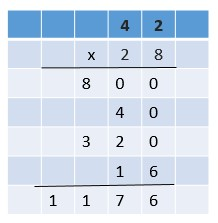So, partial products of 42 x 28 are 800, 40, 320, 16
Thus the correct answer is option c.

Spiral Review

Question 3.
Last year, the city library collected 117 used books for its shelves. This year, it collected 3 times as many books. How many books did it collect this year?
Options:
a. 832
b. 428
c. 351
d. 72

Explanation:
From the given data,
Last year, the number of used books collected by the city library by its shelves = 117 books
This year, it collected 3 times as many books = 3 × 117 =351 books
Total number of books collected by the city library for this year = 351 books
Thus the correct answer is option c.

Question 4.
Washington Elementary has 232 students. Washington High has 6 times as many students. How many students does Washington High have?
Options:
a. 1,392
b. 1,382
c. 1,292
d. 1,281

Explanation:
As per the given data,
The number of students in Washington elementary = 232 students
Washington High has 6 times as many students = 6 x 232 = 1392
Total number of students in Washington High = 1392 students
Thus the correct answer is option a.

Question 5.
What are the partial products of 35 × 7?
Options:
a. 10, 12
b. 21, 35
c. 210, 35
d. 350, 21

Explanation:
Partial products of 35 x 7 are 210, 35
Thus the correct answer is option c.

Question 6.
Shelby has ten $5 bills and thirteen$10 bills. How much money does Shelby have in all?
Options:
a. $15 b.$60
c. $63 d.$180

Answer: $180 Explanation: From the given data, Shelby has ten$5 bills and thirteen $10 bills = (10 x$5) + (13 x $10) = ($50) + ($130) =$180
Total money with Shelby = $180 Thus the correct answer is option d. ### Common Core – Multiply 2-Digit Numbers – Page No. 59 Multiply with Regrouping Estimate. Then find the product. Question 1. Estimate: 2,700 Think: 87 is close to 90 and 32 is close to 30. 90 × 30 = 2,700Question 2. 7 3 × 2 8 ——— Estimate: _______ Product: _______ Answer: Estimate: 2,100 Product: 2,044 Explanation: Estimate: 73 is close to 70; 28 is close to 30. So, 70 × 30 = 2,100. Product: Write 73 as 7 tens and 3 ones. Multiply 28 by 3 ones. 2 28 × 73 ——– 84 <– 3 × 28 Multiply 28 by 7 tens 5 28 × 73 ——– 1960 <– 70 × 28 Add the partial products. 84 + 1960 = 2,044. So, 73 × 28 = 2,044. Question 3. 4 8 × 3 8 ——— Estimate: _______ Product: _______ Answer: Estimate: 2,000 Product: 1,824 Explanation: 48 is close to 50 and 38 is close to 40. Estimate: 50 × 40 = 2,000 40 × 38 = 1520 8 × 38 = 304 1520 + 304 = 1824. Product: 1,824 Question 4. 5 9 × 5 2 ——— Estimate: _______ Product: _______ Answer: Estimate: 3,000 Product: 3,068 Explanation: 59 is close to 60 and 52 is close to 50. Estimate: 60 × 50 = 3,000 50 × 52 = 2600 9 × 52 = 468 2600 + 468 = 3068. Product: 3,068. Question 5. 8 4 × 4 0 ——— Estimate: _______ Product: _______ Answer: Estimate: 3,200 Product: 3,360 Explanation: 84 is close to 80 and 40 is close to 40. Estimate: 80 × 40 = 3,200 80 × 40 = 3,200 4 × 40 = 160 3200 + 160 = 3,360. Product: 3,360. Question 6. 8 3 × 7 7 ——— Estimate: _______ Product: _______ Answer: Estimate: 6,400 Product: 6,391 Explanation: 83 is close to 80 and 77 is close to 80. Estimate: 80 × 80 = 6,400 80 × 77 = 6,160 3 × 77 = 231 6,160 + 231 = 6,391. Product: 6,391. Question 7. 9 1 × 1 9 ——— Estimate: _______ Product: _______ Answer: Estimate: 1,800 Product: 1,729 Explanation: 91 is close to 90 and 19 is close to 20. Estimate: 90 × 20 = 1,800 90 × 19 = 1,710 1 × 19 = 19 1,710+ 19 = 1,729. Product: 1,729. Problem Solving Question 8. Baseballs come in cartons of 84 baseballs. A team orders 18 cartons of baseballs. How many baseballs does the team order? ______ baseballs Answer: 1,512 baseballs Explanation: Given, Baseballs come in cartons of 84 baseballs. A team orders 18 cartons of baseballs. To find total baseballs, 84 × 18 80 × 18 = 1,440 4 × 18 = 72 84 × 18 = 1,512 Thus the team order 1512 baseballs. Question 9. There are 16 tables in the school lunch room. Each table can seat 22 students. How many students can be seated at lunch at one time? ______ students Answer: 352 students Explanation: Given, There are 16 tables in the school lunch room. Each table can seat 22 students. Total Students = 16 × 22 10 × 22 = 220 6 × 22 = 132 220 + 132 = 352. 352 students can be seated at lunch at one time. ### Common Core – Multiply 2-Digit Numbers – Page No. 60 Lesson Check Question 1. The art teacher has 48 boxes of crayons. There are 64 crayons in each box. Which is the best estimate of the number of crayons the art teacher has? Options: a. 2,400 b. 2,800 c. 3,000 d. 3,500 Answer: 3,000 Explanation: Given, The art teacher has 48 boxes of crayons. There are 64 crayons in each box. Total number of crayons = 48 × 64 48 is close to 50; 64 is close to 60 50 × 60 = 3,000. The art teacher has about 3, 000 crayons. The correct answer is option c. Question 2. A basketball team scored an average of 52 points in each of 15 games. How many points did the team score in all? Options: a. 500 b. 312 c. 780 d. 1,000 Answer: 780 Explanation: Given, A basketball team scored an average of 52 points in each of 15 games. Total Points = 52 × 15 50 × 15 = 750 2 × 15 = 30 750 + 30 = 780. The basketball team scored 780 points in total. The correct answer is option c. Spiral Review Question 3. One Saturday, an orchard sold 83 bags of apples. There are 27 apples in each bag. Which expression represents the total number of apples sold? Options: a. 16 + 6 + 56 + 21 b. 160 + 60 + 56 + 21 c. 160 + 60 + 560 + 21 d. 1,600 + 60 + 560 + 21 Answer: 1,600 + 60 + 560 + 21 Explanation: Total number of apples sold = 83 × 27 80 × 27 = 2,160 3 × 27 = 81 2,160 + 81 = 2,241. The total number of apples sold = 2,241. 16 + 6 + 56 + 21 = 99 not equal to 2,241 160 + 60 + 56 + 21 = 297 not equal to 2,241 160 + 60 + 560 + 21 = 801 not equal to 2,241 1,600 + 60 + 560 + 21 = 2,241 equal to 2,241 1,600 + 60 + 560 + 21 = 2,241 is correct. The correct answer is option d. Question 4. Hannah has a grid of squares that has 12 rows with 15 squares in each row. She colors 5 rows of 8 squares in the middle of the grid blue. She colors the rest of the squares red. How many squares does Hannah color red? Options: a. 40 b. 140 c. 180 d. 220 Answer: 140 Explanation: Hannah has a grid of squares that has 12 rows with 15 squares in each row = 12 × 15 = 180. She colors 5 rows of 8 squares in the middle of the grid blue. The grid of squares in blue = 5 × 8 = 40. The grid of squares in red = 180 – 40 = 140. The correct answer is option b. Question 5. Gabriella has 4 times as many erasers a Leona. Leona has 8 erasers. How many erasers does Gabriella have? Options: a. 32 b. 24 c. 12 d. 2 Answer: 32 Explanation: Given, Gabriella has 4 times as many erasers a Leona. Leona has 8 erasers. Gabriella have 4 x 8 = 32 erasers. The correct answer is option a. Question 6. Phil has 3 times as many rocks as Peter. Together, they have 48 rocks. How many more rocks does Phil have than Peter? Options: a. 36 b. 24 c. 16 d. 12 Answer: 24 Explanation: Phil has 3 times as many rocks as Peter. Together, they have 48 rocks If Peter has x rocks, Phil has 3x rocks 3x + x = 48. 4x = 48. x = 48/4 = 12. Peter has 12 rocks. Phil has 3 × 12 = 36 rocks. Phil has 36 – 12 = 24 more rocks than Peter. The correct answer is option b. ### Common Core – Multiply 2-Digit Numbers – Page No. 61 Choose a Multiplication Method Estimate. Then choose a method to find the product. Question 1. Estimate: 1,200 3 1 × 4 3 ——- 9 3 + 1, 2 4 0 ———– 1, 3 3 3 Question 2. 6 7 × 8 5 ———- Estimate: _________ Product: _________ Answer: Estimate: 6,300 Product: 5,695 Explanation: Estimate: 67 is close to 70; 85 is close to 90. 70 × 90 = 6,300. Product: 67 × 85 80 × 6 tens = 480 tens 80 × 7 ones = 560 ones 5 × 6 tens = 30 tens 5 × 7 ones = 35 ones. Add partial products. 4800 + 560 + 300 + 35 = 5,695. Question 3. 6 8 × 3 8 ———- Estimate: _________ Product: _________ Answer: Estimate: 2,800 Product: 2,584 Explanation: Estimate: 68 is close to 70; 38 is close to 40. 70 × 40 = 2,800. Product: 68 × 38 30 × 6 tens = 180 tens 30 × 8 ones = 240 ones 8 × 6 tens = 48 tens 8 × 8 ones = 64 ones. Add partial products. 1800 + 240 + 480 + 64 = 2,584. Question 4. 9 5 × 1 7 ———- Estimate: _________ Product: _________ Answer: Estimate: 1,700 Product: 1,615 Explanation: Estimate: 95 is close to 100. 100 × 17 = 1,700. Product: 95 × 17 10 × 9 tens = 90 tens 10 × 5 ones = 50 ones 7 × 9 tens = 63 tens 7 × 5 ones = 35 ones. Add partial products. 900 + 50 + 630 + 35 = 1,615. Question 5. 4 9 × 5 4 ———- Estimate: _________ Product: _________ Answer: Estimate: 2,500 Product: 2,646 Explanation: Estimate: 49 is close to 50; 54 is close to 50. 50 × 50 = 2,500. Product: 49 × 54 50 × 4 tens = 200 tens 50 × 9 ones = 450 ones 4 × 4 tens = 16 tens 4 × 9 ones = 36 ones. Add partial products. 2000 + 450 + 160 + 36 = 2,646. Question 6. 9 1 × 2 6 ———- Estimate: _________ Product: _________ Answer: Estimate: 2,700 Product: 2,366 Explanation: Estimate: 91 is close to 90; 26 is close to 30. 90 × 30 = 2,700. Product: 49 × 54 20 × 9 tens = 180 tens 20 × 1 ones = 20 ones 6 × 9 tens = 54 tens 6 × 1 ones = 6 ones. Add partial products. 1800 + 20 + 540 + 6 = 2,366. Question 7. 8 2 × 1 9 ———- Estimate: _________ Product: _________ Answer: Estimate: 1,600 Product: 1,558 Explanation: Estimate: 82 is close to 80; 19 is close to 20. 80 × 20 = 1,600. Product: 82 × 19 10 × 8 tens = 80 tens 10 × 2 ones = 20 ones 9 × 8 tens = 72 tens 9 × 2 ones = 18 ones. Add partial products. 800 + 20 + 720 + 18 = 1,558. Question 8. 4 6 × 2 7 ———- Estimate: _________ Product: _________ Answer: Estimate: 1,500 Product: 1,242 Explanation: Estimate: 46 is close to 50; 27 is close to 30. 50 × 30 = 1,500. Product: 46 × 27 20 × 4 tens = 80 tens 20 × 6 ones = 120 ones 7 × 4 tens = 28 tens 7 × 6 ones = 42 ones. Add partial products. 800 + 120 + 280 + 42 = 1,242. Question 9. 4 1 × 3 3 ———- Estimate: _________ Product: _________ Answer: Estimate: 1,200 Product: 1,353 Explanation: Estimate: 41 is close to 40; 33 is close to 30. 40 × 30 = 1,200. Product: 41 × 33 30 × 4 tens = 120 tens 30 × 1 ones = 30 ones 3 × 4 tens = 12 tens 3 × 1 ones = 3 ones. Add partial products. 1200 + 30 + 120 + 3 = 1,353. Question 10. 9 7 × 1 3 ———- Estimate: _________ Product: _________ Answer: Estimate: 1,300 Product: 1,261 Explanation: Estimate: 97 is close to 100. 100 × 13 = 1,300. Product: 97 × 13 10 × 9 tens = 90 tens 10 × 7 ones = 70 ones 3 × 9 tens = 27 tens 3 × 7 ones = 21 ones. Add partial products. 900 + 70 + 270 + 21 = 1,261. Question 11. 7 5 × 6 9 ———- Estimate: _________ Product: _________ Answer: Estimate: 5,600 Product: 5,195 Explanation: Estimate: 75 is close to 80; 69 is close to 70. 80 × 70 = 5,600. Product: 75 × 69 60 × 7 tens = 420 tens 60 × 5 ones = 300 ones 9 × 7 tens = 63 tens 9 × 5 ones = 45 ones. Add partial products. 4200 + 300 + 630 + 45 = 5,195. Problem Solving Question 12. A movie theatre has 26 rows of seats. There are 18 seats in each row. How many seats are there in all? _____ seats Answer: 468 seats Explanation: Given, A movie theatre has 26 rows of seats. There are 18 seats in each row. 26 x 18 = 468 seats. 20 x 18 = 360 6 x 18 = 108 108+360 = 468. Therefore there are 468 seats in all. Question 13. Each class at Briarwood Elementary collected at least 54 cans of food during the food drive. If there are 29 classes in the school, what was the least number of cans collected? _____ cans Answer: 1,566 cans Explanation: Each class at Briarwood Elementary collected at least 54 cans of food. If there are 29 classes in the school, the least number of cans collected = 54 x 29 = 1,566 cans. ### Common Core – Multiply 2-Digit Numbers – Page No. 62 Lesson Check Question 1. A choir needs new robes for each of its 46 singers. Each robe costs$32. What will be the total cost for all 46 robes?
Options:
a. $1,472 b.$1,372
c. $1,362 d.$230

Answer: $1,472 Explanation: Given, A choir needs new robes for each of its 46 singers. Each robe costs$32.
46 × $32 40 ×$32 = $1,280 6 ×$32 = $192$1,280 + $192 =$1,472
The correct answer is option a.

Question 2.
A wall on the side of a building is made up of 52 rows of bricks with 44 bricks in each row. How many bricks make up the wall?
Options:
a. 3,080
b. 2,288
c. 488
d. 416

Explanation:
Given,
A wall on the side of a building is made up of 52 rows of bricks with 44 bricks in each row.
52 × 44
50 × 44 = 2,200
2 × 44 = 88
2,200 + 88 = 2,288.
2,288 bricks make up the wall.
The correct answer is option b.

Spiral Review

Question 3.
Which expression shows how to multiply 4 × 362 by using place value and expanded form?
Options:
a. (4 × 3) + (4 × 6) + (4 × 2)
b. (4 × 300) + (4 × 600) +(4 × 200)
c. (4 × 300) + (4 × 60) + (4 × 20)
d. (4 × 300) + (4 × 60) + (4 × 2)

Answer: (4 × 300) + (4 × 60) + (4 × 2)

Explanation:
4 × 362 = 1,448
a. (4 × 3) + (4 × 6) + (4 × 2) = 12 + 24 + 8 = 44 not equal to 1,448.
b. (4 × 300) + (4 × 600) +(4 × 200) = 1200 + 2400 + 800 = 4,400 not equal to 1,448.
c. (4 × 300) + (4 × 60) + (4 × 20) = 1200 + 240 + 80 = 1,520 not equal to 1,448.
d. (4 × 300) + (4 × 60) + (4 × 2) = 1200 + 240 + 8 = 1,448 equal to 1,448.
The correct answer is option d.

Question 4.
Use the model below. What is the product 4 × 492?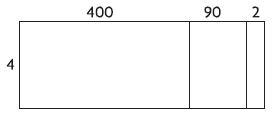Options:
a. 16 + 36 + 8 = 60
b. 160 + 36 + 8 = 204
c. 160 + 360 + 8 = 528
d. 1,600 + 360 + 8 = 1,968

Answer: 1,600 + 360 + 8 = 1,968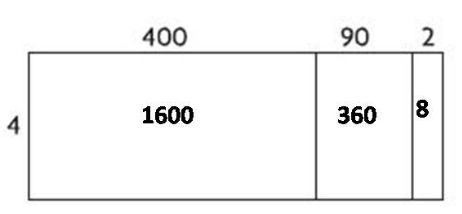1,600 + 360 + 8 = 1,968
The correct answer is option d.

Question 5.
What is the sum 13,094 + 259,728?
Options:
a. 272,832
b. 272,822
c. 262,722
d. 262,712

Explanation:
13,094 + 259,728 = 262,722
The correct answer is option c.

Question 6.
During the 2008–2009 season, there were 801,372 people who attended the home hockey games in Philadelphia. There were 609,907 people who attended the home hockey games in Phoenix. How much greater was the home attendance in Philadelphia than in Phoenix that season?
Options:
a. 101,475
b. 191,465
c. 201,465
d. 202,465

Explanation:
Given,
During the 2008–2009 season, there were 801,372 people who attended the home hockey games in Philadelphia.
There were 609,907 people who attended the home hockey games in Phoenix.
801,372 – 609,907 = 191,465
Philadelphia attendance is 191,465 greater than in Phoenix that season.
The correct answer is option b.

### Common Core – Multiply 2-Digit Numbers – Page No. 63

Problem Solving Multiply 2 – Digit numbers

Solve each problem. Use a bar model to help.

Question 1.
Mason counted an average of 18 birds at his bird feeder each day for 20 days. Gloria counted an average of 21 birds at her bird feeder each day for 16 days. How many more birds did Mason count at his feeder than Gloria counted at hers?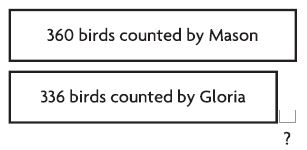Birds counted by Mason: 18 × 20 = 360
Birds counted by Gloria: 21 × 16 = 336
Draw a bar model to compare.
Subtract. 360 – 336 = 24
So, Mason counted 24 more birds.

Question 2.
The 24 students in Ms. Lee’s class each collected an average of 18 cans for recycling. The 21 students in Mr. Galvez’s class each collected an average of 25 cans for recycling. How many more cans were collected by Mr. Galvez’s class than Ms. Lee’s class?
_____ more cans

Explanation:
The number of cans collected by Ms. Lee’s class = 18 × 24 = 432.
The number of cans collected by Mr. Galvez’s class = 25 × 21 = 525.
Use Bar ModelSubtract. 525 – 432 = 93 more cans.
So, Mr. Galvez’s class collected 93 more cans than Ms. Lee’s class.

Question 3.
At East School, each of the 45 classrooms has an average of 22 students. At West School, each of the 42 classrooms has an average of 23 students. How many more students are at East School than at West School?
_____ more students

Explanation:
Students in East school = 45 × 22 = 990.
Students in West School = 42 × 23 = 966.
Use Bar Model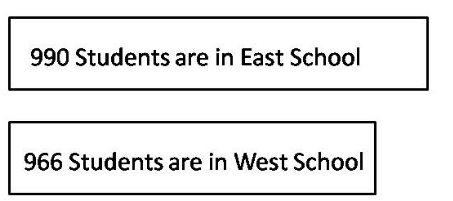Subtract. 990 – 966 = 24.
So, East School has 24 students more than West School.

Question 4.
A zoo gift shop orders 18 boxes of 75 key rings each and 15 boxes of 80 refrigerator magnets each. How many more key rings than refrigerator magnets does the gift shop order?
_____ more key rings

Explanation:
Number of Key Rings = 75 x 18 = 1,350.
Number of Refrigerator Magnets= 80 x 15 = 1,200.
Use Bar Model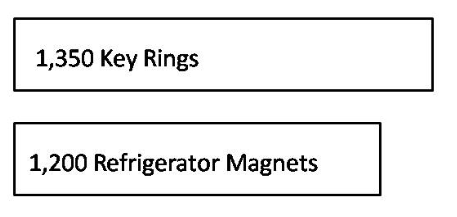Subtract. 1,350 – 1,200 = 150.
So, key rings are 150 more than refrigerator magnets.

### Common Core – Multiply 2-Digit Numbers – Page No. 64

Lesson Check

Question 1.
Ace Manufacturing ordered 17 boxes with 85 ball bearings each. They also ordered 15 boxes with 90 springs each. How many more ball bearings than springs did they order?
Options:
a. 5
b. 85
c. 90
d. 95

Explanation:
Number of ball bearings = 85 × 17 = 1,445.
Number of springs = 90 × 15 = 1,350.
Use Bar ModelSubtract. 1,445 – 1,350 = 95.
So, ball bearings are 95 more than springs.
The correct answer is option d.

Question 2.
Elton hiked 16 miles each day on a 12-day hiking trip. Lola hiked 14 miles each day on her 16-day hiking trip. In all, how many more miles did Lola hike than Elton hiked?
Options:
a. 2 miles
b. 18 miles
c. 32 miles
d. 118 miles

Explanation:
Hiking trip by Elton = 12 × 16 = 192.
Hiking trip by Lola = 16 × 14 = 224.
Use Bar ModelSubtract. 224 – 192 = 32.
So, the Hiking trip by Lola is 32 times more than the Hiking trip by Elton.
The correct answer is option c.

Spiral Review

Question 3.
An orchard has 24 rows of apple trees. There are 35 apple trees in each row. How many apple trees are in the orchard?
Options:
a. 59
b. 192
c. 740
d. 840

Explanation:
An orchard has 24 rows of apple trees. There are 35 apple trees in each row.
24 × 35 = 840 apple trees are in the orchard.
The correct answer is option d.

Question 4.
An amusement park reported 354,605 visitors last summer. What is this number rounded to the nearest thousand?
Options:
a. 354,600
b. 355,000
c. 360,000
d. 400,000

Explanation:
An amusement park reported 354,605 visitors last summer. 4,605 is close to 5,000.
The correct answer is option b.

Question 5.
Attendance at the football game was 102,653. What is the value of the digit 6?
Options:
a. 6
b. 60
c. 600
d. 6,000

Explanation:
Digit 6 is at hundreds of positions.
So, the answer is 6 x 100 = 600.
The correct answer is option c.

Question 6.
Jill’s fish weighs 8 times as much as her parakeet. Together, the pets weigh 63 ounces. How much does the fish weigh?
Options:
a. 7 ounces
b. 49 ounces
c. 55 ounces
d. 56 ounces

Explanation:
Let Jill’s parakeet = X.
Jill’s fish weighs 8 times as much as her parakeet = 8X.
Together, the pets weigh 63 ounces.
X + 8X = 63.
9X = 63.
X = 63/9 = 7.
So, Jill’s parakeet =7.
Jill’s fish = 7 × 8 = 56 ounces.
The correct answer is option d.

### Common Core – Multiply 2-Digit Numbers – Page No. 65

Lesson 3.1

Choose a method. Then find the product.

Question 1.
12 × 60 = _____

Explanation:
By using the place value method
You can take 10 as 1 ten
12 × 60 = 12 × 6 ten
= 72 ten
12 × 60 = 720

Question 2.
56 × 40 = _____

Explanation:
56 × 40
By using the associative property
You can think of 40 as (4 × 10)
56 × 40 = 56 × (4 × 10)
= (56 × 4) × 10
= 224 × 10
56 × 40 = 2240

Question 3.
30 × 40 = _____

Explanation:
30 × 40
By using the place value method
You can take 30 as 3 tens
30 × 40 = 30 × 4 tens
= 120 tens
30 × 40 = 1200

Question 4.
50 × 67 = _____

Explanation:
By using the place value method
You can take 50 as 5 tens
50 x 67 = 67 x 5 tens
= 335 tens
50 × 67 = 3350

Lesson 3.2

Estimate the product. Choose a method.

Question 5.
33 × 76 = _____

Round to the nearest tens.
33 is close to 30; 76 is close to 80;
Use the mental math
30 × 80  = 2400
So, estimated product of 33 × 76 = 2400

Question 6.
43 × 90 = _____

Explanation:
Round to the nearest tens.
43 is close to 40; 90 is close to 90;
Use the mental math, then
9 × 4 = 36
90 × 40 = 3,600
So, estimated product of 43 × 90 = 3,600

Question 7.
47 × $66 = _____ Answer:$3500

Explanation:
Round to the nearest tens.
47 is close to 50; 66 is close to 70;
Use the mental math, then
50 × 70 = 3,500
So, estimated product of 50 × 70 = $3,500 Question 8. 12 × 81 = _____ Answer: 800 Explanation: Round to the nearest tens. 12 is close to 10; 81 is close to 80; Use mental math, then 10 × 80 = 800 So, the estimated product of 12 × 81 = 800 Question 9. 46 × 47 = _____ Answer: 2500 Explanation: Round to the nearest tens. 46 is close to 50; 47 is close to 50; Use mental math, then 50 × 50 = 2500 So, the estimated product of 46 × 47 = 2500 Question 10. 58 × 79 = _____ Answer: 4800 Explanation: Round to the nearest tens. 58 is close to 60; 79 is close to 80; Use mental math, then 60 × 80 = 4800 So, the estimated product of 58 × 79 = 4800 Question 11. 24 × 73 = _____ Answer: 1400 Explanation: Round to the nearest tens. 24 is close to 20; 73 is close to 70; Use mental math, then 20 × 70 = 1400 So, the estimated product of 24 × 73 = 1400 Question 12. 68 × 36 = _____ Answer: 2800 Explanation: Round to the nearest tens. 68 is close to 70; 36 is close to 40; Use mental math, then 70 × 40 = 2800 So, the estimated product of 68 × 36 = 2800 Lesson 3.3 Draw a model to represent the product. Then record the product. Question 13. 41 × 16 = _____ Answer: 656 Explanation: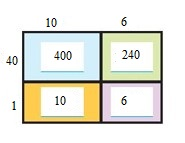400 + 240 + 10 + 6 = 656 Question 14. 39 × 52 = _____ Answer: 2028 Explanation:1500 + 60 + 450 + 18 = 2028 Question 15. 94 × 36 = _____ Answer: 3384 Explanation:2700 + 540 + 120 + 24 = 3384 ### Common Core – Multiply 2-Digit Numbers – Page No. 66 Lesson 3.4 Record the product. Question 1. 5 3 × 3 7 ——— ________ Answer: 1961Question 2. 4 8 × 47 ——— ________ Answer: 2256Question 3. 6 5 × 2 8 ——— ________ Answer: 1820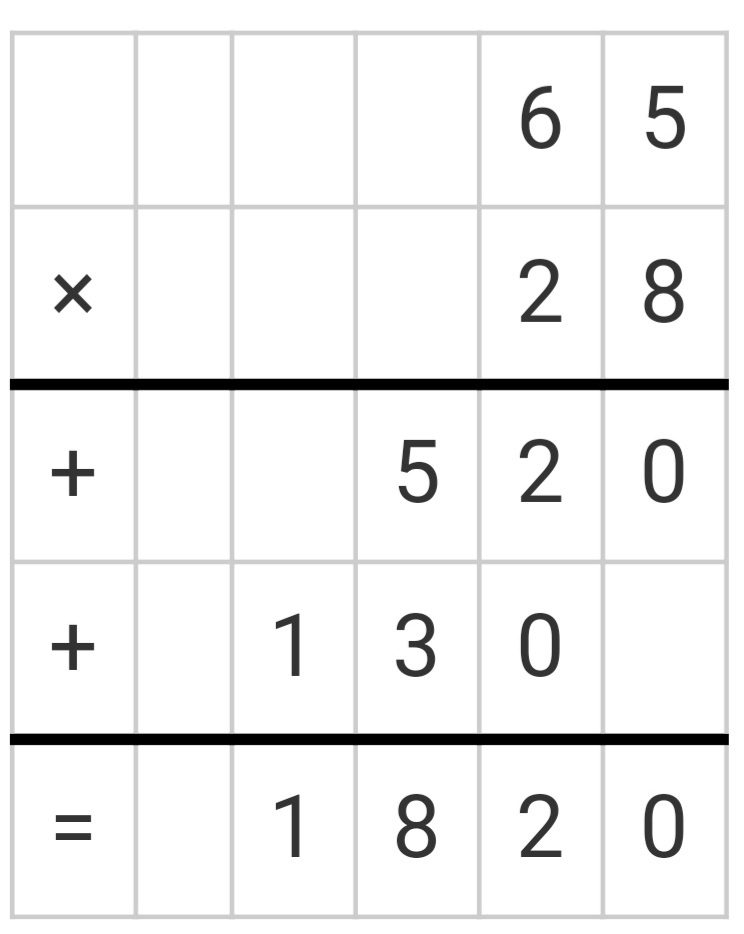Question 4. 9 2 × 7 9 ——— ________ Answer: 7268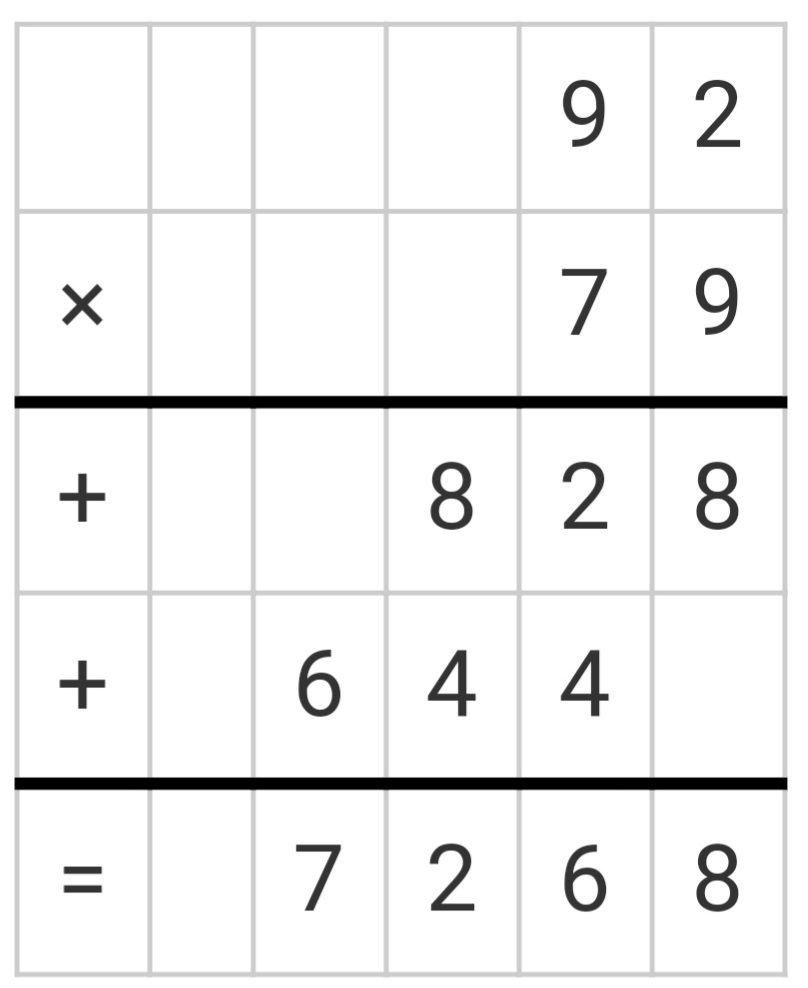Lessons 3.5–3.6 Estimate. Then choose a method to find the product. Question 5. 4 8 × 2 1 ——— Estimate: ________ Product: ________ Answer: Explanation: 48 × 21 Estimate: Think 48 is close to 50; 21 is close to 20. 50 x 20 = 1000 Product: 10 x 5 tens = 50 tens 50 x 2 ones = 100 ones 2 x 2 tens = 40 tens 4 x 2 ones = 8 ones. Add partial products. 500 + 100 + 400 + 8 = 1008. Question 6.$ 7 2
× 4 6
———
Estimate: ________
Product: ________

Estimate: Think 72 is close to 70; 46 is close to 50.
70 × 50 = 3500
Product:
70 x 4 tens = 280 tens
50 x 2 ones = 100 ones
4 x 10 tens = 40 tens
4 x 6 ones = 24 ones.
2800 + 100 + 400 + 12 = 3312

Question 7.
$3 9 × 5 8 ——— Estimate: ________ Product: ________ Answer: 2262 Estimate: Think 39 is close to 40; 58 is close to 60. 40 × 60 = 2400 Product: 30 x 5 tens = 150 tens 9 x 8 ones = 72 ones 8 x 3 tens = 24 tens 9 x 5 tens = 45 tens. Add partial products. 1500 + 240 + 450 + 72 = 2262 Question 8. 27 ×$ 19
Estimate: ________
Product: ________

Estimate: Think 27 is close to 30; 19 is close to 20.
30 × 20 = 600
Product:
20 x 9 ones = 180 ones
9 x 7 ones = 63 ones
20 x 1 tens = 20 tens
7 x 10 ones = 70 ones.
180 + 70 + 63 + 200 = 513

Question 9.
97 × 32
Estimate: ________
Product: ________

Estimate: Think 97 is close to 100; 32 is close to 30.
100 × 30 = 3000
Product:
30 x 9 tens = 270 tens
30 x 7 ones = 210 ones
2 x 9 tens = 18 tens
2 x 7 ones = 14 ones.
2700 + 210 + 14 + 180 = 3104

Question 10.
44 × 69
Estimate: ________
Product: ________

Estimate: Think 44 is close to 40; 69 is close to 70.
100 × 30 = 3000
Product:
40 x 6 tens = 240 tens
40 x 9 ones = 360 ones
4 x 6 tens = 24 tens
4 x 9 ones = 36 ones.
2400 + 360 + 240 + 36 = 3036

Question 11.
Last week, Ms. Simpson worked 28 hours. She stocked shelves for 45 minutes each hour for 14 of those hours. The rest of the time she worked in customer service. How many minutes last week did Ms. Simpson work in customer service? (Hint: 1 hour = 60 minutes)
______ minutes

Explanation:
Given,
Last week, Ms. Simpson worked 28 hours.
She stocked shelves for 45 minutes each hour for 14 of those hours.
The rest of the time she worked in customer service.
45 × 14 = 630
She worked 630 minutes stocking shelves, which equals 10 hours and 30 minutes.
After that, she worked another 17 hours and 30 minutes in customer service.
17 × 60 = 1020
1020 + 30 = 1050
Ms. Simpson worked 1050 minutes in customer service.

Question 12.
The after-school craft center has 15 boxes of 64 crayons each. In 12 of the boxes, 28 of the crayons have not been used. All the rest have been used. How many of the crayons in the center have been used?
______ crayons